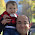## Monday, March 4, 2013

### Basic Function Types in Tableau

Today, we will talk about the different basic functions in Tableau.  This was prompted by a poster on the Tableau forums asking for more information about these functions.  I am not an expert on Tableau's data engine.  Therefore, I will keep this discussion somewhat high-level.

SQL FUNCTIONS

These functions all exist in the SQL language.  It is my hypothesis that they are passed directly to the underlying SQL for computational efficiency.  However, this is one area I am currently looking into.  If anyone knows for sure, please let me know in the comments.

COUNT( [Any Row-Level Data Type] )

This function returns the number of non-null rows in the pane.  If you have a complete data set, this will be identical to SUM( 1 ).  This function is useful if you want to know how many rows satisfy a certain condition.  For example, you can find the number of rows from 2013 with

IF [Year] = 2013 THEN COUNT( [Year] ) END

SUM( [Row-Level Numeric] )

This function returns the sum of non-null rows in the pane.  I believe it to be the easiest function to comprehend.  For example, you can find total sales with

SUM( [Sales] )

AVG( [Row-Level Numeric] )

This function returns the average of non-null rows in the pane.  Care should be taken when working with this function.  It returns the sum of all non-null rows divided by the count of all non-null rows.  If you want an average calculated at a level that isn't the row-level, you need to create your own SUM() and COUNT() functions.  For example, you can find average profit with

AVG( [Profit] )

COUNTD( [Any Row-Level Data Type] )

This function returns the distinct count of non-null rows in the pane.  It is especially useful for calculating averages at a different grain than your underlying data.  If my data was at the transaction level, I could calculate the average profit per store as

SUM( [Profit] ) / COUNTD( [StoreNumber] )

DATEADD( [Date Unit], [Integer], [Date] )

This function adds a certain number of units to a date value.  The unit type and number of units are supplied by the user.  For example, 3 days after [Date] can be found as

DATEADD( "day", 3, [Date] )

DATEDIFF( [Date Unit], [Start Date], [End Date] )

This function returns the number of date units between the start and end dates.  For example, the number of years between [Date1] and [Date2] is

DATEDIFF( "year", [Date1], [Date2] )

LEN( [String] )

This function returns the number of characters in a string.  For example, the number of characters in "Tableau" ( 7 ) is

LEN( "Tableau" )

LEFT( [String], [Integer] )

This function returns the first [Integer] characters in a string.  For example, the first 4 characters in "Tableau" (Tabl) is

LEFT( "Tableau", 4 )

TABLEAU FUNCTIONS

To my knowledge, these functions do not have direct relationships to SQL functions.  Therefore, my hypothesis is that these are calculated within Tableau.  If there is more to this, please let me know in the comments.

LOOKUP( [Function], [Offset] )

This is the workhorse of all window functions.  It returns the value of the given function at another value outside of the current pane.  This requires use of "Compute Using."  If you are not familiar with "Compute Using", please refer to my previous post Working with Table Calculations in Tableau.  For example, if you want to the see the value of SUM( [Sales] ) in the previous row, you can use

LOOKUP( SUM( [Sales] ), -1 )

RUNNING_SUM( [Function] )

This function returns the sum of the function in all previous rows in the table.  For example, the sum of all previous daily counts is

RUNNING_SUM( COUNT( [Sales] ) )

WINDOW_SUM( [Function], { [StartOffset], [EndOffset] } )

This function returns the sum of the function for all rows within the window defined by [StartOffset] and [EndOffset].  This is the first occurrence of optional parameters.  If [StartOffset] and [EndOffset] are omitted, the entire table is used.  For example, if you would like to the sum of sales for the last 3 rows (4 rows total including the current row), you can use

WINDOW_SUM( SUM( [Sales] ), -3, 0 )

FIRST()

This function returns the current offset from the first row.  For example, if you want to emulate RUNNING_SUM using WINDOW_SUM, you can use

WINDOW_SUM( SUM( [Sales] ), FIRST(), 0 )

These are a good portion of the Tableau functions that I regularly use.  However, there are quite a few useful functions that are not mentioned here.  If you feel like I missed something important, let me know.  I hope you found this informative.  Thanks for reading.

Associate Consultant
Mariner, LLC
llewellyn.wb@gmail.com

1.Tableau actually compiles calculated field formulas into SQL expressions in most cases. Table Calculations are one of the exceptions, as are operations involving locally computed values such as filtering by the geocoded, auto-generated Latitude and Longitude fields.
-Robert

1.Robert,

Thanks for the info. I've actually been meaning to write a new post about functions now that I'm much more experienced with the tool.

Cheers!

2.I am learning Tableau from the basics up. I have a solid background in Microsoft SQL Server. Where would you add the functions listed above into a Tableau table? I.E. I am trying to count a number of distinct businessentityID's and have it return them in column grouped by the State/Province they are within.